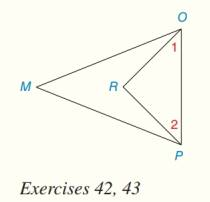Chapter 1.CR, Problem 43CRElementary Geometry for College St...

6th Edition
Daniel C. Alexander + 1 other
ISBN: 9781285195698

Solutions

Chapter
SectionElementary Geometry for College St...

6th Edition
Daniel C. Alexander + 1 other
ISBN: 9781285195698
Textbook Problem

Write two-column proofs for Review Exercises 39 to 46. Given: ∠ M O P ≅ ∠ M P O O R → bisects ∠ M O P P R → bisects ∠ M P O Prove: ∠ 1 ≅ ∠ 2To determine

To find:

The two-column proof for 12.

Explanation

Given:

The given figure is,

Figure (1)

MOPMPO, OR bisects MOP, PR bisects MPO.

Approach:

An angle bisector splits an angle into two equal angles.

The reasons for given statements is explained in table below.

 Statements Reasons 1. ∠MOP≅∠MPO 1. Given 2. OR→ bisects ∠MOP 2. Given 3. PR→ bisects ∠MPO 3

Still sussing out bartleby?

Check out a sample textbook solution.

See a sample solution

The Solution to Your Study Problems

Bartleby provides explanations to thousands of textbook problems written by our experts, many with advanced degrees!

Get Started

Explain the difference between a matched-subjects design and a repeated-measures design.

Essentials of Statistics for The Behavioral Sciences (MindTap Course List)

Find f'(x) and f"(x). f(x)=x21+ex

Single Variable Calculus: Early Transcendentals, Volume I

In Exercises 73-80, find the indicated limits, if they exist. 78. limx4x43x2+12x4+x3+x2+x+1

Applied Calculus for the Managerial, Life, and Social Sciences: A Brief Approach

Convert the expressions in Exercises 6584 to power form. 4x23+x3/2623x2

Finite Mathematics and Applied Calculus (MindTap Course List)

Test the series for convergence or divergence. 10. n=1n2en3

Single Variable Calculus: Early Transcendentals

In Exercises 110, find the graphical solution to each inequality. 3y+50

Finite Mathematics for the Managerial, Life, and Social Sciences

Which is the best graph of y = 1.5x? (The graph of y = 2x is shown as a dashed curve for comparison.)

Study Guide for Stewart's Single Variable Calculus: Early Transcendentals, 8th